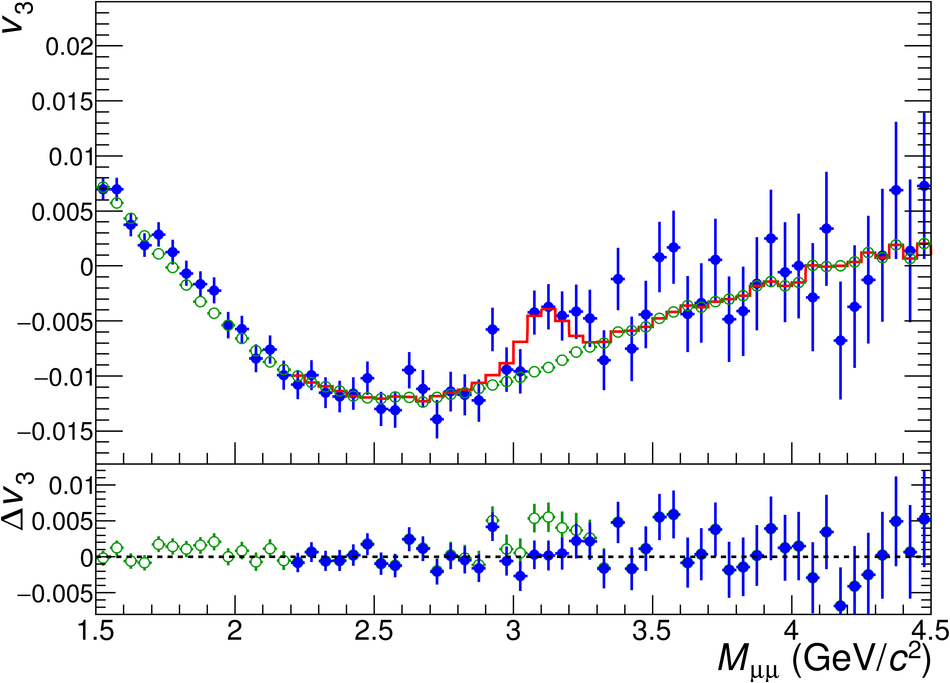# Figure 3

 The $v_2(M_{\mu\mu})$ (left panel) and $v_3(M_{\mu\mu})$ (right panel) distributions in the 0--50\ are fitted with the function from Eq.~\ref{eq:vn_sb}, where the background coefficients $v_2^{\rm B}(M_{\mu\mu})$ and $v_3^{\rm B}(M_{\mu\mu})$ are fixed using the event-mixing procedure. The background coefficients alone down to 1.5 GeV/$c^2$ are also presented. Only statistical uncertainties are shown.